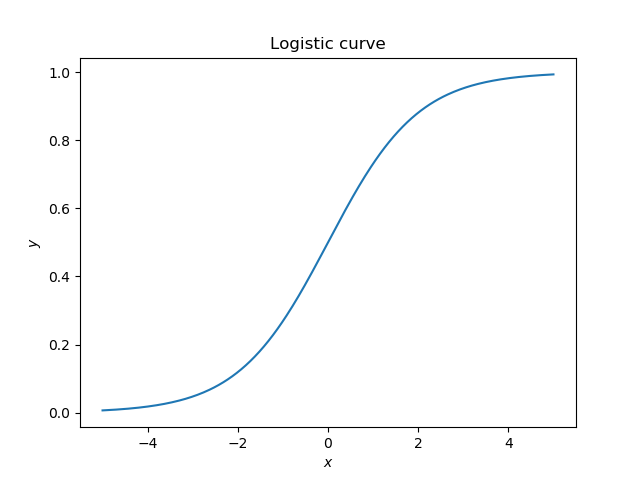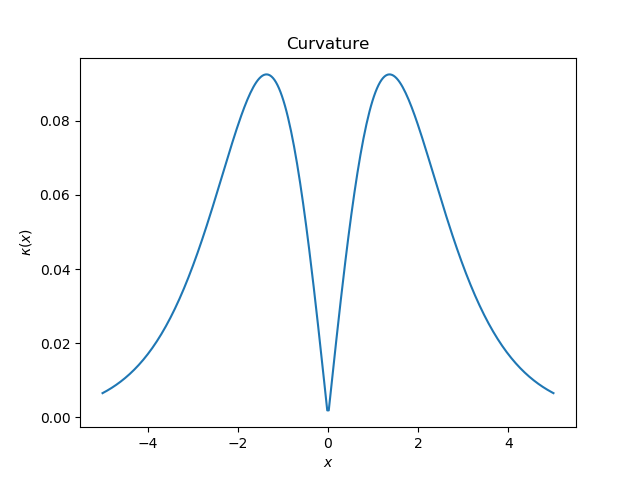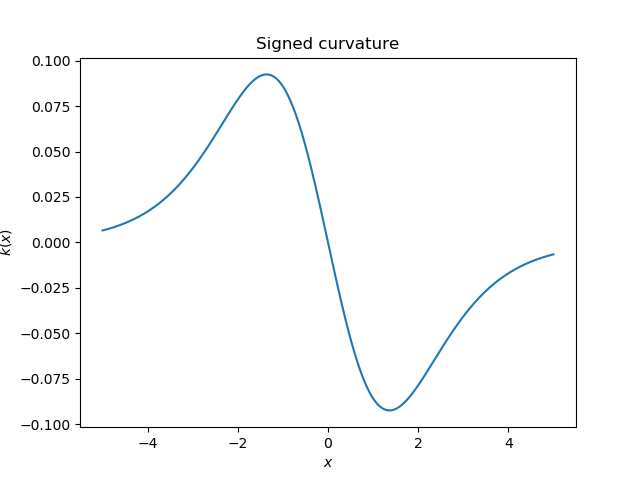## Python 实现

$$y=\frac{1}{1+e^{-x}}$$

$$\kappa (x)=\frac{|y^{‘’}|}{(1+y^{‘2})^{3/2}}$$

## 绘图$$k(x) = \frac{y^{‘’}}{(1+y^{‘2})^{3/2}}$$